Practice
Resources
Contests
Online IDE
New
Free Mock
Scaler
Practice
Improve your coding skills with our resources
Contests
Compete in popular contests with top coders
Scaler
Explore Offerings by SCALER## Welcome to Interviewbit, help us create the best experience for you!

Currently, You are a:College/University *
Enter the name of your college
Branch *
Year of completion *College/University *
Enter the name of your college
Branch *
Year of completion *Current Company *
Enter company name
Experience *## You're all set!Full name *
Email *

By creating an account, I acknowledge that I have read and agree to InterviewBit’s Terms and Privacy Policy .## Welcome back!

Email *Go to Problems

Be a Code Ninja!

# Dijkstra algorithm

The algorithm was developed by a Dutch computer scientist Edsger W. Dijkstra in 1956. It is used to find the shortest path between a node/vertex (source node) to any (or every) other nodes/vertices (destination nodes) in a graph. A graph is basically an interconnection of nodes connected by edges. This algorithm is sometimes referred to as Single Source Shortest Path Algorithm due to its nature of implementation.

Table of content

### Application

#### Working of Dijkstra Algorithm

Before diving into any algorithm, its very much necessary for us to understand what are the real world applications of it. Majority of the problems that we encounter in real life scenarios deals with finding solutions to shortest path based problems. To explain in simple words, you want to travel from city A to city B. Both cities are connected by multiple routes. What route do we generally prefer? There is no doubt that we would opt for the route which can make us reach our destination with minimum possible cost and time! Isn’t this relatable?
Following are the main applications of Dijkstra’s Algorithm:

1. It is most widely used in finding shortest possible distance and show directions between 2 geographical locations such as in Google Maps.
2. This is also widely used in routing of data in networking and telecommunication domains for minimizing the delay occurred for transmission.
3. Wherever you encounter the need for shortest path solutions be it in robotics, transportation, embedded systems, factory or production plants to detect faults, etc this algorithm is used.

### Explanation

#### Working of Dijkstra's Shortest Path First algorithm

In order to find the shortest path, Dijkstra’s algorithm mainly allocates a “cost” value taken to reach the destination vertex from the source vertex. The “cost” can be mapped to disance, money or time taken to reach from source to a destination.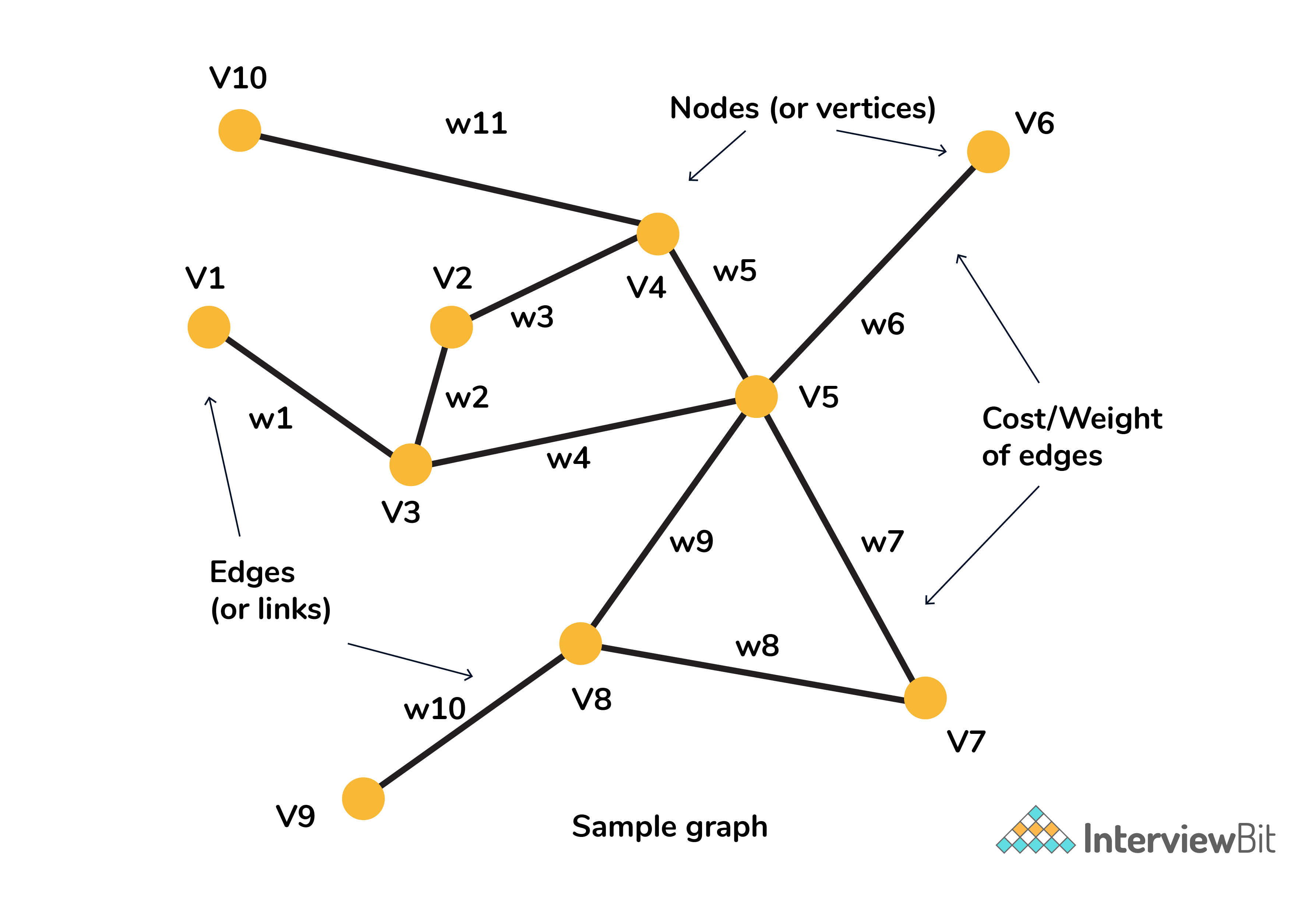Below are the steps to be followed for solving using Dijkstra’s algorithm:

1. Convert any problem to its graph equivalent representation.
2. Maintain a list of unvisited vertices. Assign a vertex as “source” and also allocate a maximum possible cost (infinity) to every other vertex. The cost of the source to itself will be zero as it actually takes nothing to go to itself.
3. In every step of the algorithm, it tries to minimize the cost for each vertex.
4. For every unvisited neighbor (V2, V3) of the current vertex (V1) calculate the new cost from V1.
5. The new cost of V2 is calculated as :
Minimum( existing cost of V2 , (sum of cost of V1 + the cost of edge from V1 to V2) )

1. When all the neighbors of the current node are visited and cost has been calculated, mark the current node V1 as visited and remove it from the unvisited list.
2. Select next vertex with smallest cost from the unvisited list and repeat from step 4.
3. The algorithm finally ends when there are no unvisited nodes left.

### Dijkstra's algorithm example

#### Problem statement in shortest path

Consider the map below. The cities have been selected and marked from alphabets A to F and every edge has a cost associated with it.
We need to travel from Bengaluru to all other places and we have to identify what are the shortest paths with minimal cost from Bengaluru to other destinations.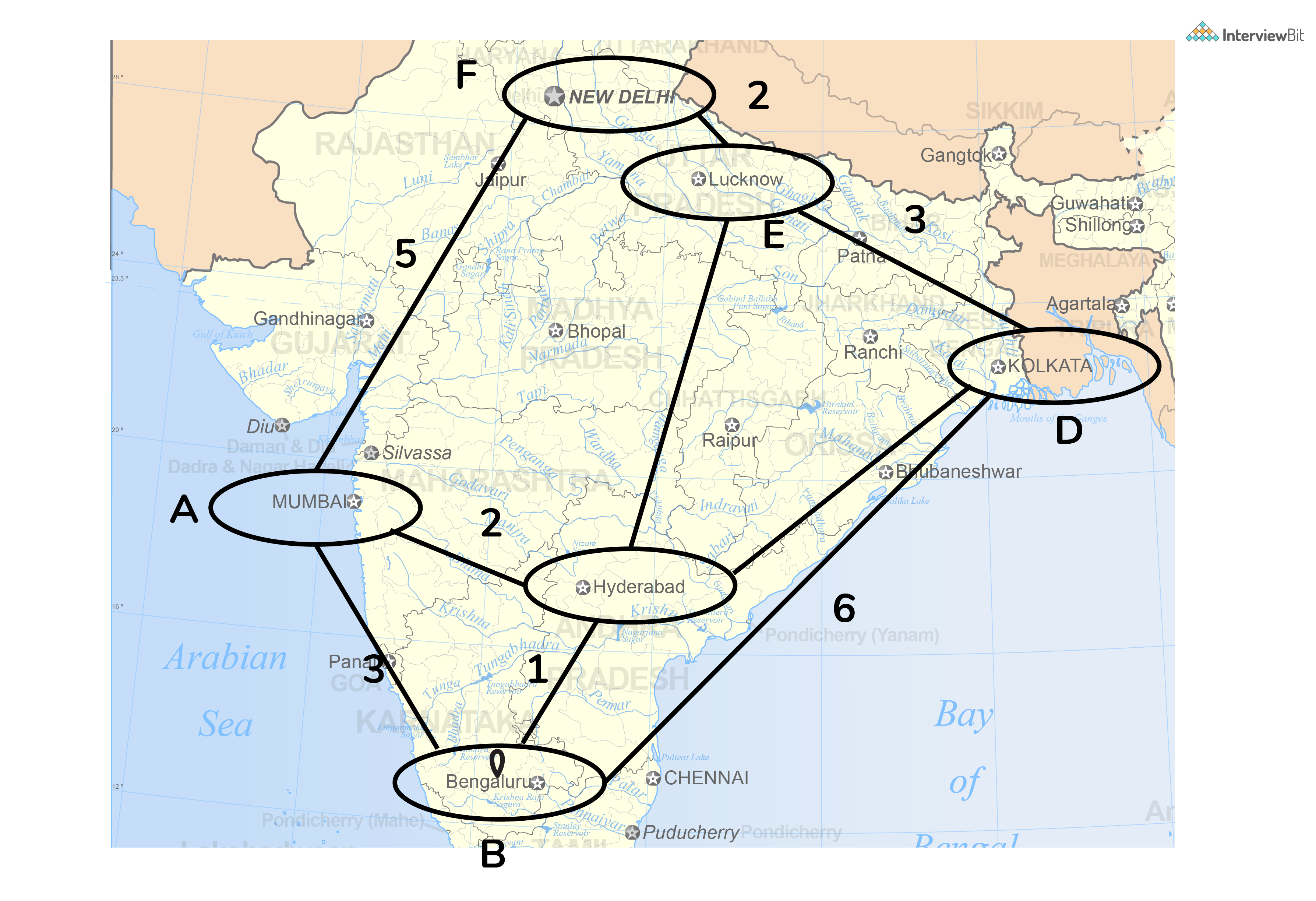#### 1. Convert problem to its graph equivalent.

Upon conversion, we get the below representation. Note that the graph is weighted and undirected. All the cities have been replaced by the alphabets associated with it and the edges have the cost value (to go from one node to other) displayed on it.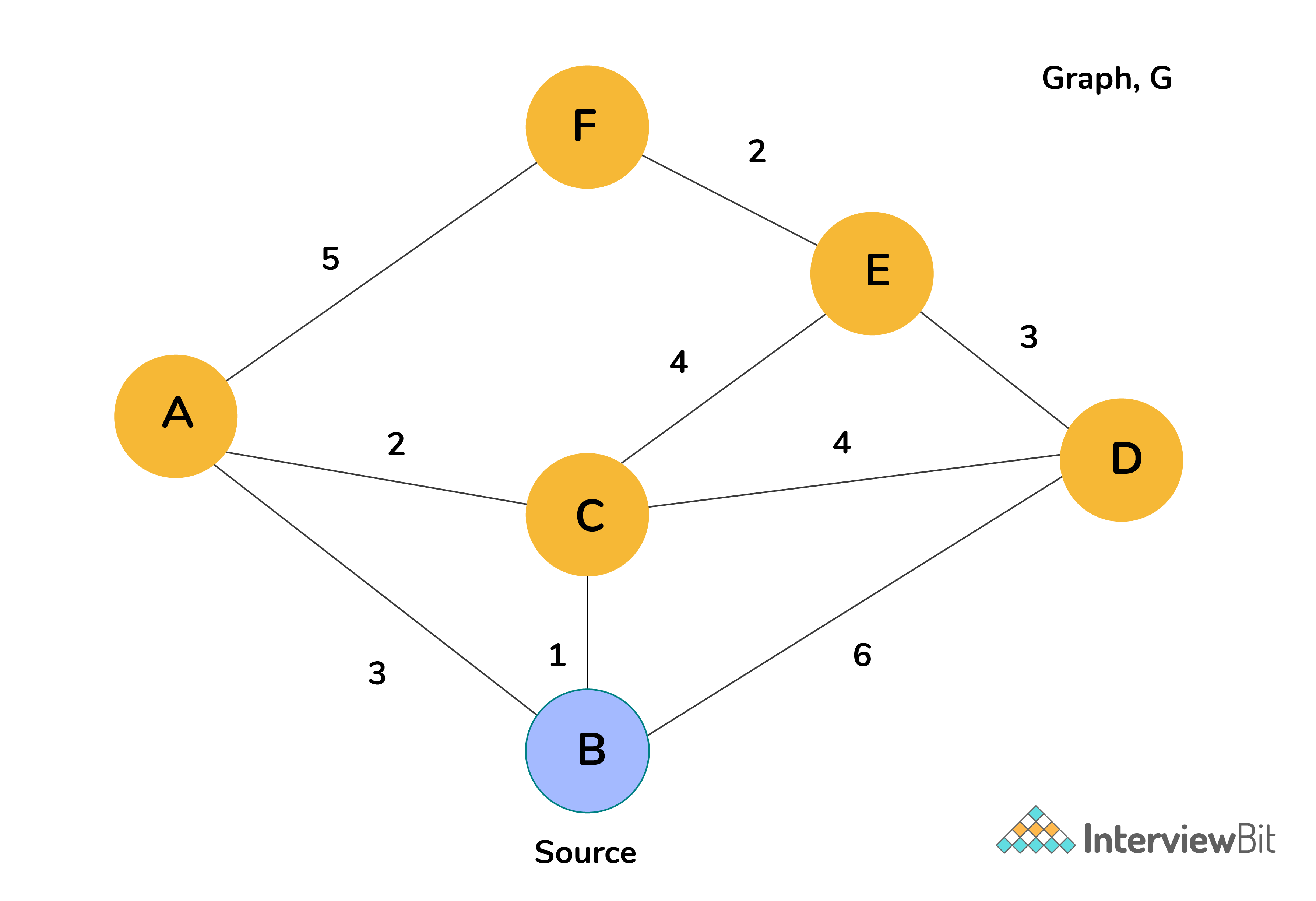#### 2. Assign cost to vertices.

Assign cost of 0 to source vertex and  (Infinity) to all other vertices as shown in the image below.
Maintain a list of unvisited vertices. Add all the vertices to the unvisted list.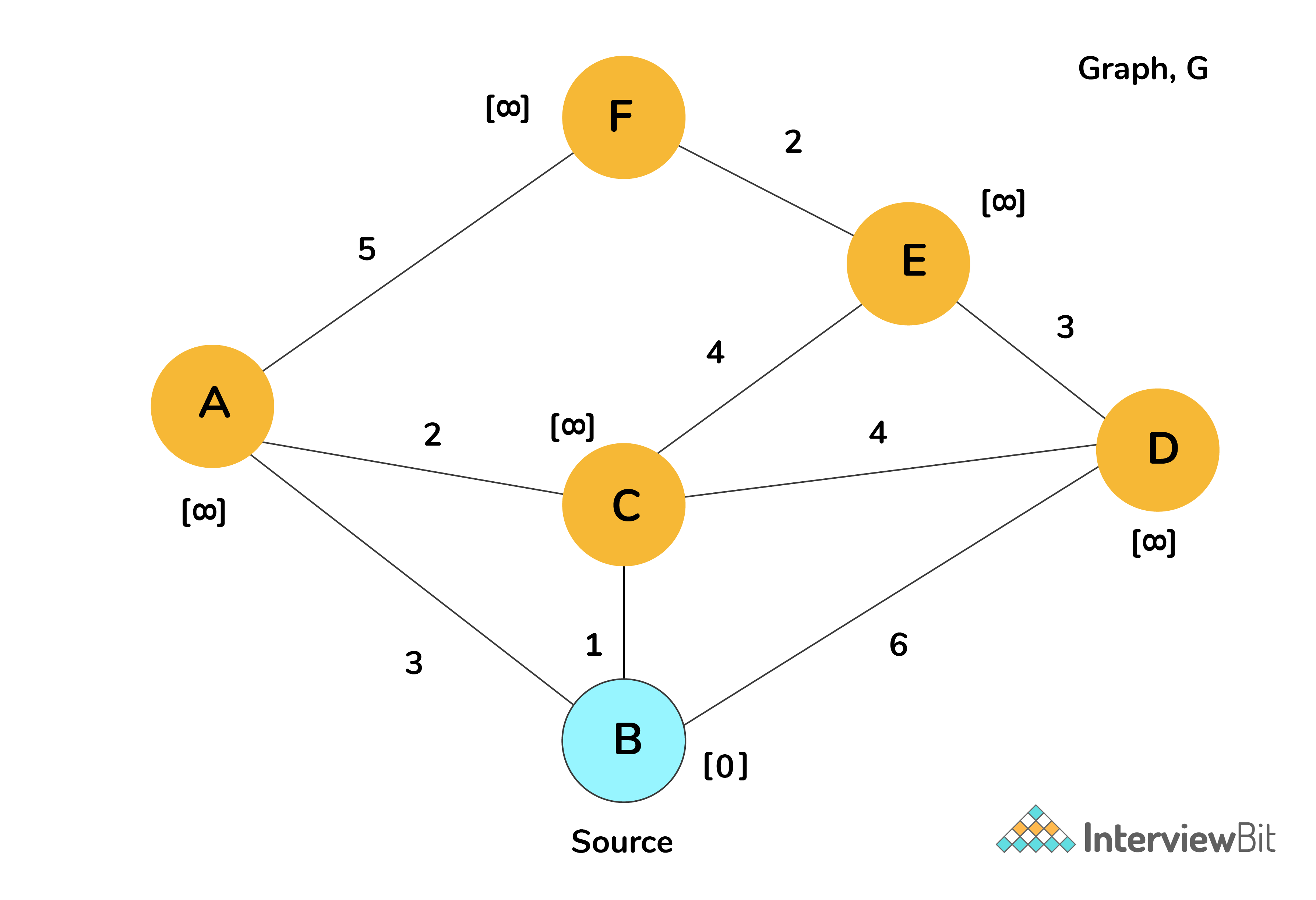#### 3. Calculate minimum cost for neighbors of selected source.

For each neighbor A, C and D of source vertex selected (B), calculate the cost associated to reach them from B using the formula. Once this is done, mark the source vertex as visited (The vertex has been changed to blue to indicate visited).

Minimum(current cost of neighbor vertex, cost(B)+edge_value(neighbor,B))

1. For neighbor A: cost = Minimum( , 0+3) = 3
2. For neighbor C: cost = Minimum( , 0+1) = 1
3. For neighbor D: cost = Minimum( , 0+6) = 6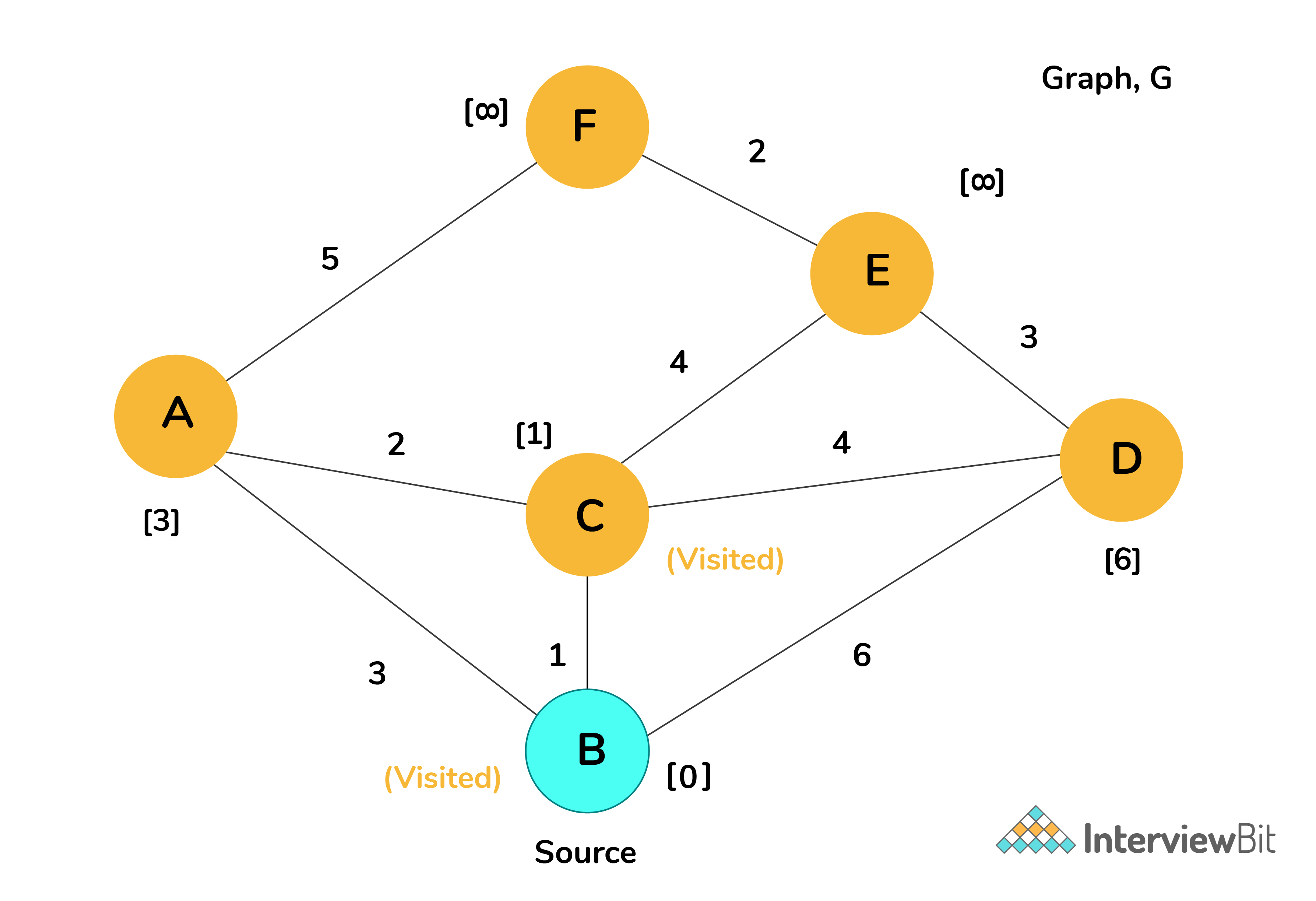#### 4. Select next vertex with smallest cost from the unvisited list.

Choose the unvisited vertex with minimum cost (here, it would be C) and consider all its unvisited neighbors (A,E and D) and calculate the minimum cost for them.

Once this is done, mark C as visited.

Minimum(current cost of neighbor vertex, cost(C)+edge_value(neighbor,C))

1. For neighbor A: cost = Minimum(3 , 1+2) = 3
2. For neighbor E: cost = Minimum(, 1+4) = 5
3. For neighbor D: cost = Minimum(6 , 1+4) = 5

Observe that the cost value of node D is updated by the new minimum cost calculated.#### 5. Repeat step 4 for all the remaining unvisited nodes.

Repeat step 4 until there are no unvisited nodes left. At the end of the execution, we will know the shortest paths from the source vertex B to all the other vertices. The final state of the graph would be like below.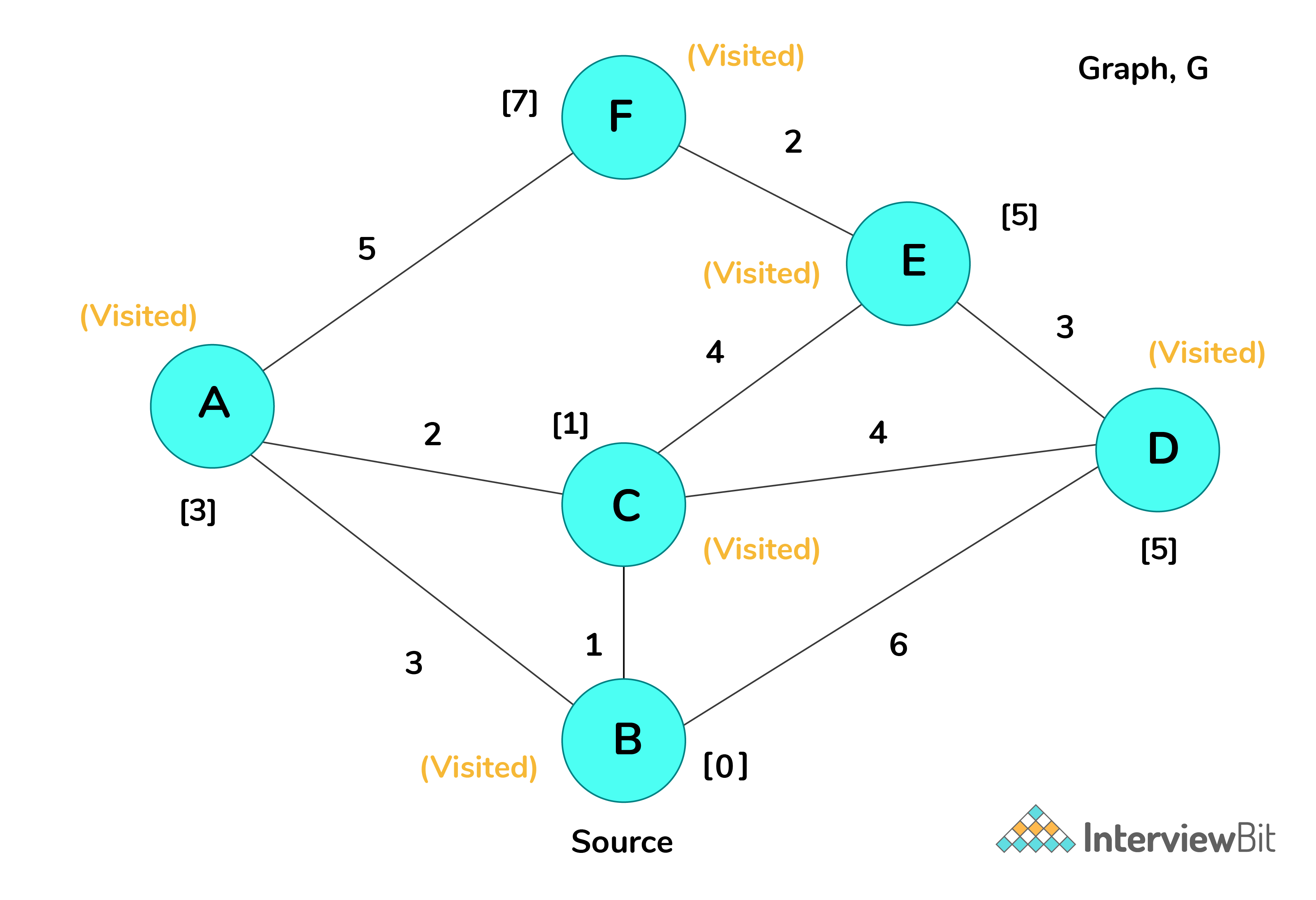### Pseudo Code of Dijkstra algorithm

Dijkstra_Algorithm(source, G):
"""
parameters: source node--> source, graph--> G
return: List of cost from source to all other nodes--> cost
"""
unvisited_list = []			// List of unvisited verticesvertices
cost = []
cost[source] = 0              // Distance (cost) from source to source will be 0
for each vertex v in G:       // Assign cost as INFINITY to all vertices
if v ≠ source
cost[v] = INFINITY
add v to unvisited_list    // All nodes pushed to unvisited_list initially

while unvisited_list is not empty:        	     // Main loop
v = vertex in unvisited_list with min cost[v]      // v is the source node for first iteration
remove v from unvisited_list		            // Marking node as visited

for each neighbor u of v:			// Assign shorter path cost to neigbour u
cost_value = Min( cost[u], cost[v] + edge_cost(v, u)]
cost[u] = cost_value            		// Update cost of vertex u

return cost

### Complexity Analysis of Dijsktra algorithm

Consider there are V number of vertices in a graph. Then by definition, there would be |V-1| number of edges.

1. The main outer loop runs for |V| times
2. The inner loop meant where actual cost calculation happens, runs for |V-1| times for a complete graph as each vertex has |V-1| edges.
3. Also, for each iteration of the inner loop we do an extractMin and a decreaseKey operation for the vertex.

Hence the total running time will have an upper bound of O(|V| * |V-1|) which is equivalent to O(|V|2)

#### Note

1. We can further reduce the time complexity of this algorithm by using Binary Heap as data structure for Priority Queue implementation instead of list.
2. The priority queue implementation is for efficiently finding the node with minimum cost and then updating the cost value associated with the node.
3. With this, the time complexity will be O((E+V)*LogV) = O(ELogV) where E is the number of edges and V is the number of vertices in a graph

### Proof of Correctness

How can we be sure that Dijkstra’s algorithm provides us the shortest possible path between two nodes? Let’s go through the following explanation to understand whether this algorithm always gives us the shortest possible path.
Consider the following notations:

D(s,u) = the minimum distance as calculated by Dijkstra's algorithm between nodes s and u
d(s,u) = the actual minimum distance between nodes s and u


According to Dijkstra’s Algorithm, D(s,u) = d(s,u)

Proof:

• Let us start by assuming that Dijkstra’s Algorithm is incorrect.

• This means there would be some vertices left when a vertex u is included into the Visited List which indicates => D(s,u) > d(s,u)
• Let x be the first of these vertices that was pushed into the Visited List. Then,

• When node x is included into the Visited List: D(s,x) > d(s,x)
• This implies that all previous vertices, say z that were included into the Visited List signifies D(s,z) = d(s,z)
• Let’s look at the moment when this node x is included into the Visited List.
• Let P be the (real) shortest path from s to x
• Let z be the first node that is not in the “Visited List” and is along the shortest path.
• Let y be the predecessor node of z on the shortest path P
• Have a look at the diagram below for better understanding: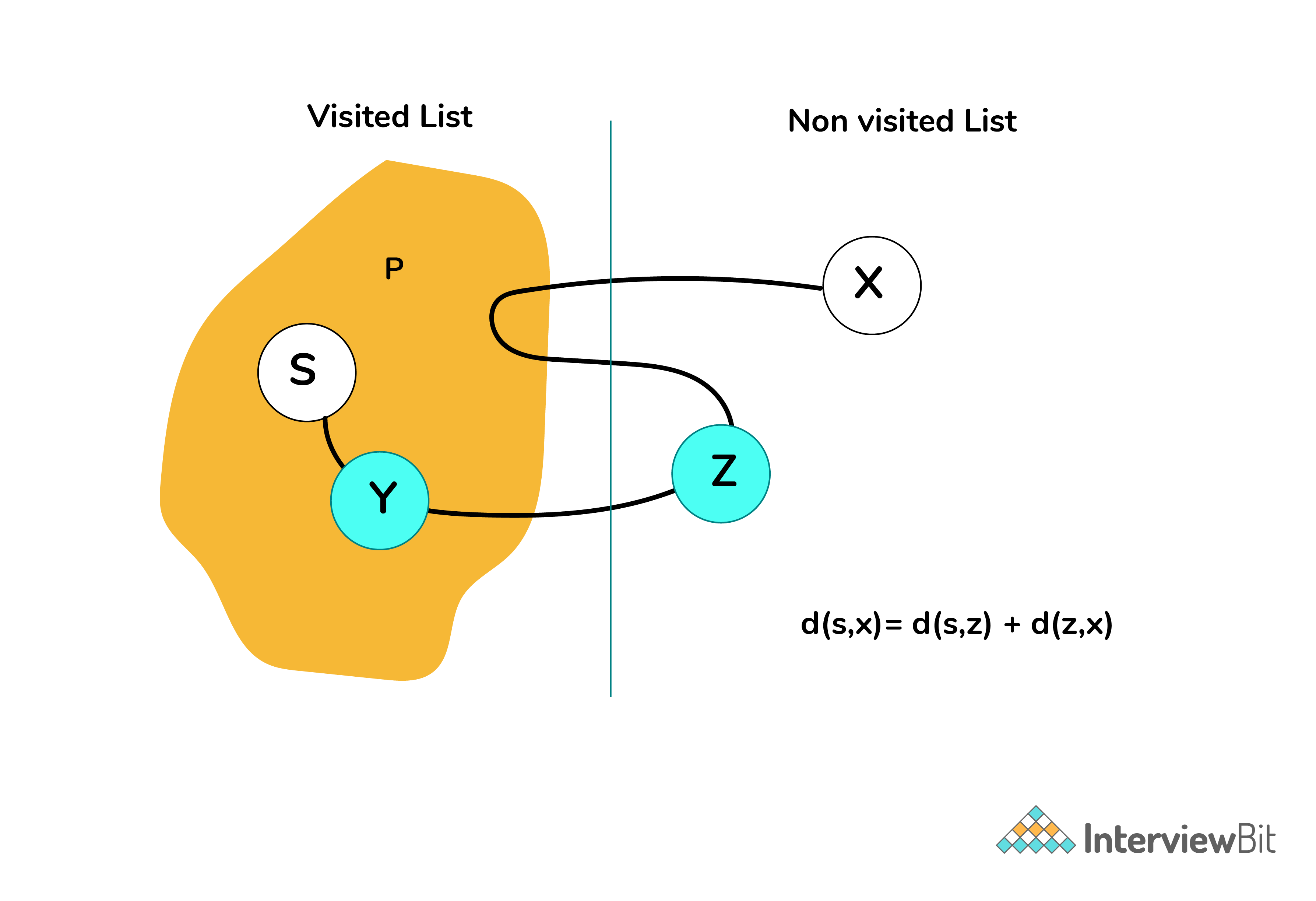• We can conclude the following:
• D(s,y) = d(s,y)  … (1) (This means the minimum distance s to y computed by the algorithm = actual min. distance s to y as y is included before x)
• D(s,z) = D(s,y) + edge_cost(y,z) = d(s,y) + edge_cost(y,z) … (2)
• D(s,x) ≤ D(s,z) …(3) (Because the next vertex included by the algorithm is x)
• We can now finally deduce that:

     D(s,x) ≤ D(s,z)                        ... From (3)
= d(s,y) + edge_cost(y,z)        ... From (2)

≤ d(s,y) + edge_cost(y,z) + d(z,x)

= d(s,x)

• The above result contradicts the assumed fact of Dijkstra’s algorithm being incorrect earlier.

• Hence, by proof of contradiction, we can say that Dijkstra’s algorithm always gives us the shortest possible path between 2 nodes which is: D(s,x) should be equal to d(s,x)

1. The example demo was done for undirected graph. However, Dijkstra’s Algorithm can also be used for directed graphs as well.
2. The pseudo code finds the shortest path from source to all other nodes in the graph. If we want it to be from a source to a specific destination, we can break the loop when the target is reached and minimum value is calculated.
3. The shortest path might not pass through all the vertices. Also, there can be more than one shortest path between two nodes.
4. Dijkstra’s Algorithm doesnt work for graphs with negative edges. Algorithms like Bellman-Ford Algorithm will be used for such cases.

## Serious about Learning Programming ?

Learn this and a lot more with Scaler Academy's industry vetted curriculum which covers Data Structures & Algorithms in depth.

## Graph Data Structure & Algorithms Problems

Graph traversal
Problem Score Companies Time Status
Path in Directed Graph 150 40:20
Water Flow 200
69:12
Stepping Numbers 300 59:52
Capture Regions on Board 500
65:57
Word Search Board 500 59:16
Depth first search
Problem Score Companies Time Status
Path with good nodes! 150 57:16
Largest Distance between nodes of a Tree 200
77:39
Good Graph 200
61:47
Cycle in Directed Graph 200 55:20
Delete Edge! 200 63:57
Two teams? 300 52:28
Bfs
Problem Score Companies Time Status
Valid Path 200 83:46
Region in BinaryMatrix 200 44:12
Level Order 300 29:55
Smallest Multiple With 0 and 1 300 74:26
Min Cost Path 300 65:56
Permutation Swaps! 300 55:45
Graph connectivity
Problem Score Companies Time Status
Convert Sorted List to Binary Search Tree 300
44:30
Shortest path
Problem Score Companies Time Status
Sum Of Fibonacci Numbers 300
52:49
Knight On Chess Board 300 67:27
Useful Extra Edges 400 62:08
Graph hashing
Problem Score Companies Time Status
Clone Graph 500 56:55

Problem Score Companies Time Status
Connected Components 200 19:55
File Search 200 20:42
Mother Vertex 200 50:15
Path in Matrix 200 26:20Free Mock Assessment
Help us know you better for the best experience
Current Employer *
Enter company name